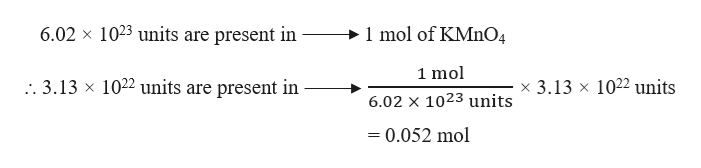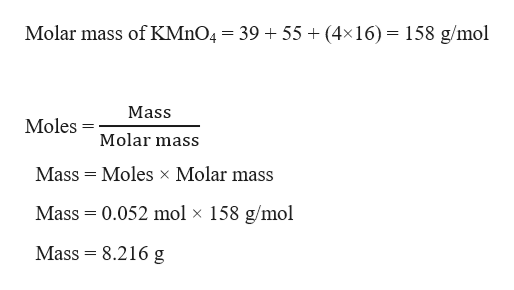# how many grams of KMnO4correspond to 3.13 x 1022 formula units of KMnO4? Avogrado's number is 6.02 x 1023

Question

how many grams of KMnO4correspond to 3.13 x 1022 formula units of KMnO4? Avogrado's number is 6.02 x 1023

check_circle

Step 1

Given,

Formula units of KMnO4 = 3.13 × 1022

Avogadro’s number = 6.02 × 1023

Moles in 3.13 x 1022 formula units of KMnO4 can be calculated as:help_outlineImage Transcriptionclose6.02 x 1023 units are present in 1 mol of KMnO4 1 mol :. 3.13 x 1022 units are present in x 3.13 x 1022 units 6.02 x 1023 units = 0.052 mol fullscreen
Step 2

Grams of KMnO4 in 0.052 mol can ...help_outlineImage TranscriptioncloseMolar mass of KMnO4 = 39 + 55 (4x16) = 158 g/mol Mass Moles Molar mass Mass Moles x Molar mass Mass 0.052 mol x 158 g/mol Mass 8.216 g fullscreen

### Want to see the full answer?

See Solution

#### Want to see this answer and more?

Solutions are written by subject experts who are available 24/7. Questions are typically answered within 1 hour.*

See Solution
*Response times may vary by subject and question.
Tagged in

### General Chemistry